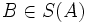# Seminormal subgroup

This article defines a subgroup property: a property that can be evaluated to true/false given a group and a subgroup thereof, invariant under subgroup equivalence. View a complete list of subgroup properties[SHOW MORE]
This is a variation of normality|Find other variations of normality | Read a survey article on varying normality
This is a variation of permutability|Find other variations of permutability |

## History

The term seminormal subgroup has many equivalent definitions. The one given here is due to Xiang Ying Su.

## Definition

### Definition with symbols

A subgroup$A$ of a finite group$G$ is termed seminormal if there exists a subgroup$B$ such that$AB = G$ and for any proper subgroup$C$ of$B$,$AC$ is a proper subgroup of$G$.

Such a$B$ is termed a S-supplement to$A$. The set of all S-supplements of a group$A$ is denoted as$S(A)$.

## Facts

### The set of S-supplements is closed under conjugacy

It turns out that if$B \in S(A)$ then so does$B^x$ for any$x \in G$. This result was proved by Su in 1988. Template:Fill-proof

## Relation with other properties

### Stronger properties

These properties are stronger in the case of finite groups:

### Relation with simplicity

In a simple group, any proper nontrivial seminormal subgroup must be a subgroup of prime index.